Function Repository Resource:

Obtain wavelength-dependent solar irradiance data for the Earth

Contributed by: Jason Martinez
 ResourceFunction["SolarIrradianceData"][type,λ] gives solar irradiance data of the type type for wavelength λ. ResourceFunction["SolarIrradianceData"][type,f] gives solar irradiance data for frequency f. ResourceFunction["SolarIrradianceData"][type,{λ1,λ2}] gives the total solar irradiance within a wavelength range from λ1 to λ2. ResourceFunction["SolarIrradianceData"][type,{f1,f2}] gives the total solar irradiance within a frequency range from f1 to f2. ResourceFunction["SolarIrradianceData"][type,format] gives full solar irradiance data in the specified format.

## Details

Solar irradiance is the spectral irradiance and provided in units of watts per square meter (of illuminated surface) per nm of wavelength or simply watts per square meter per nanometer. This is typical for solar irradiance data, such as the source, the 2000 ASTM Standard Extraterrestrial Spectrum Reference and similar products.
For pairs of wavelengths, solar irradiance over the wavelengths and returned in units of watts per square meter.
Frequency input is converted to wavelengths to find the irradiance or spectral irradiance. For a single frequency results are returned in units of watts per square meter per hertz. Pairs of frequencies are integrated like wavelengths with results in watts per square meter.
ResourceFunction["SolarIrradianceData"][] can be used to get a list of supported type values. Available values for type are:
Wavelengths and frequencies should be given as Quantity expressions.
Quantities of intervals are integrated over like pairs of wavelengths or frequencies.
The value of format in ResourceFunction["SolarIrradianceData"][type,format] can be "Data" (the default) or "Interpolation". "Data" returns a QuantityArray of available data while "Interpolation" returns an InterpolatingFunction with the available data.

## Examples

### Basic Examples (3)

Calculate the solar irradiance of 500 nanometers light on the Earth's surface:

 In:=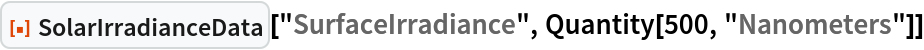Out=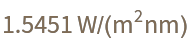Determine the solar irradiance of 500 THz light on the uppermost reaches of the Earth's atmosphere:

 In:=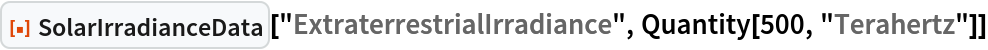Out=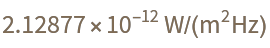Compute the solar irradiance for green light on the earth's surface:

 In:=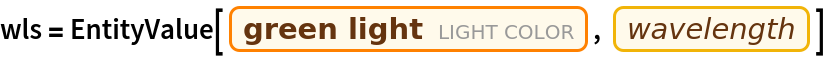Out=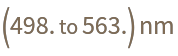In:=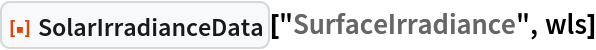Out=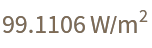### Scope (3)

Specify frequencies to integrate solar irradiance over:

 In:=Out=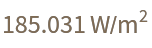Obtain all the data for surface solar irradiance:

 In:=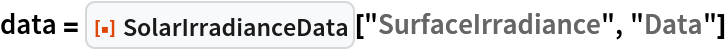Out=Find the wavelength in the data set with the maximum solar irradiance:

 In:=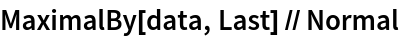Out=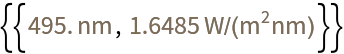Retrieve an interpolating function for solar irradiance outside the atmosphere:

 In:=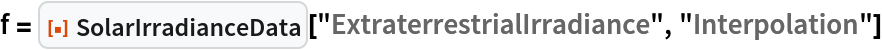Out=In:=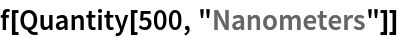Out=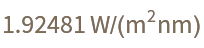### Applications (1)

Compare solar irradiance above and below the atmosphere:

 In:=Out=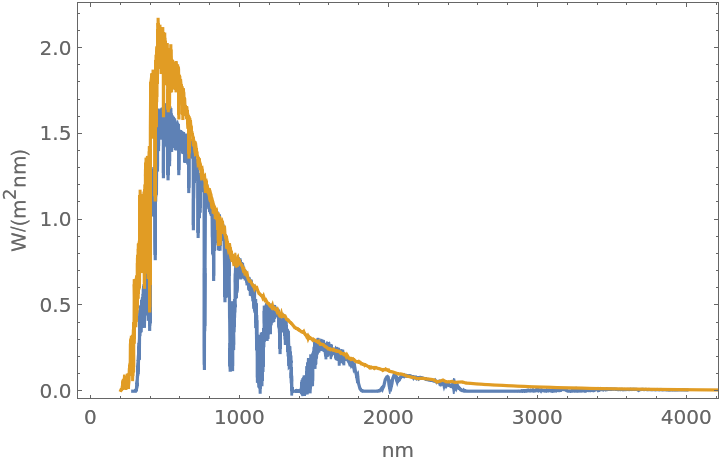### Properties and Relations (1)

Compare the peak wavelength in the data set for extraterrestrial irradiance to that predicted for a blackbody:

 In:=Out=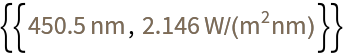In:=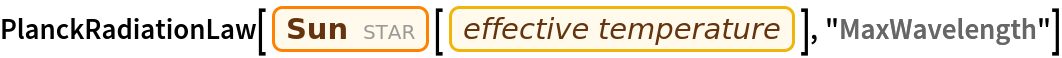Out=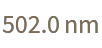## Version History

• 1.0.0 – 25 October 2022